Courses

# Irodov Solutions: Wave Properties of Particles. Schrodinger Equation- 1 Notes | EduRev

## JEE : Irodov Solutions: Wave Properties of Particles. Schrodinger Equation- 1 Notes | EduRev

The document Irodov Solutions: Wave Properties of Particles. Schrodinger Equation- 1 Notes | EduRev is a part of the JEE Course I. E. Irodov Solutions for Physics Class 11 & Class 12.
All you need of JEE at this link: JEE

Q.49. Calculate the de Broglie wavelengths of an electron, proton, and uranium atom, all having the same kinetic energy 100 eV.

Ans. The kinetic eneigy is nonrelativistic in all three cases. Now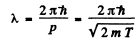using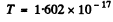Joules, we get

λ= 122.6 pm

λp = 2.86 pm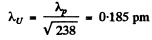(where we have used a mass number of 238 for the U nucleus).

Q.50. What amount of energy should be added to an electron to reduce its de Broglie wavelength from 100 to 50 pm?

Ans. From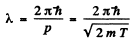we find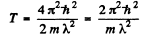Thus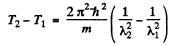Substitution gives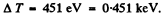Q.51. A neutron with kinetic energy T = 25 eV strikes a stationary deuteron (heavy hydrogen nucleus). Find the de Broglie wavelengths of both particles in the frame of their centre of inertia.

Ans. We shall use M0 = 2Mn. The CM is moving with velocity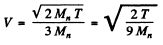with respect to the Lab frame. In the CM frame the velocity of neutron is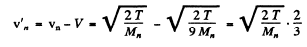and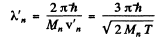Substitution gives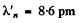Since the momenta are equal in the CM frame the de Broglie wavelengths will also be equal. If we do not assume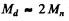we shall get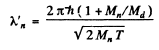Q.52. Two identical non-relativistic particles move at right angles to each other, possessing de Broglie wavelengths λ1 and λ2. Find the de Broglie wavelength of each particle in the frame of their centre of inertia.

Ans. If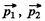are the momenta o f the two particles then their momenta in the CM frame will be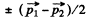as the particle are identical.

Hence their de Broglie wavelength will be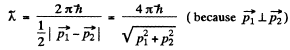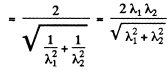Q.53. Find the de Broglie wavelength of hydrogen molecules, which corresponds to their most probable velocity at room temperature.

Ans. In thermodynamic equilibrium, Maxwell’s velocity distribution law holds :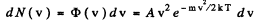φ (v) is maximum when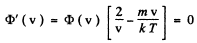The difines the most probable velocity,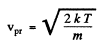The de Broglie wavelength of H molecules with the most probable velocity is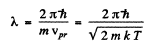Substituting the appropriate value especially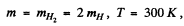we get

λ = 126 pm

Q.54. Calculate the most probable de Broglie wavelength of hydrogen molecules being in thermodynamic equilibrium at room temperature.

Ans.  To find the most probable de Broglie wavelength of a gas in thermodynamic equilibrium we determine the distribution is λ corresponding to Maxwellian velocity distribution.
It is given by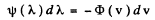(where - sign takes account of the fact that λ decreaes as v increases). Now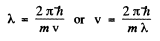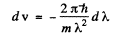Thus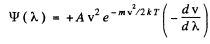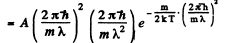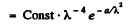where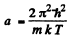This is maximum when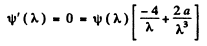or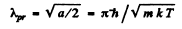Using the result of the previous problem it is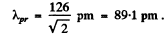Q.55. Derive the expression for a de Broglie wavelength λ of a relativistic particle moving with kinetic energy T. At what values of T does the error in determining λ using the non-relativistic formula not exceed 1% for an electron and a proton?

Ans. For a relativistic particle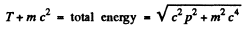Squaring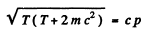Hence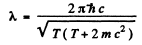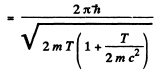If we use nonrelativistic formula,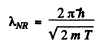SO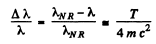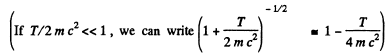Thus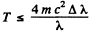i,f the error is less than Δλ

For electron the error is not more than 1 % if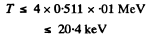For a proton, the error is not more than 1 % if

T ≤ 4 X 938 X 0.01 MeV
i.e.                T ≤ 37.5 MeV.

Q.56. At what value of kinetic energy is the de Broglie wavelength of an electron equal to its Compton wavelength?

Ans. The de Broglie wavelength is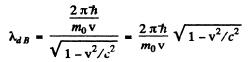and the Compton wavelength is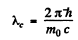The two are equal if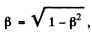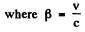or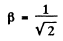The corresponding kinetic energy is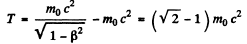Here mis th rest mass of the particle (here an electron).

Q.57. Find the de Broglie wavelength of relativistic electrons reaching the anticathode of an X-ray tube if the short wavelength limit of the continuous X-ray spectrum is equal to λsh = 10.0 pm?

Ans.  For relativistic electrons, the formula for the short wavelength limit of X - rays will be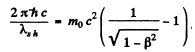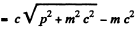or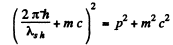or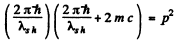or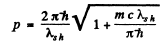Hence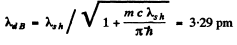Q.58. A parallel stream of monoenergetic electrons falls normally on a diaphragm with narrow square slit of width b = 1.0 μm. Find the velocity of the electrons if the width of the central diffraction maximum formed on a screen located at a distance l = 50 cm from the slit is equal to Δx = 0.36 mm.

Ans. he first minimum in a Fraunhofer diffraction is given by (b is the width of the slit)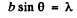Here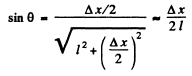Thus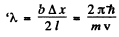so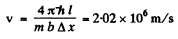Q.59. A parallel stream of electrons accelerated by a potential difference V = 25 V falls normally on a diaphragm with two narrow slits separated by a distance d = 50μm. Calculate the distance between neighbouring maxima of the diffraction pattern on a screen located at a distance l = 100 cm from the slits.

Ans.  From the Young slit foimula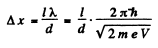Substitution gives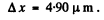Q.60. A narrow stream of monoenergetic electrons falls at an angle of incidence θ = 30° on the natural facet of an aluminium single crystal. The distance between the neighbouring crystal planes parallel to that facet is equal to d = 0.20 nm. The maximum mirror reflection is observed at a certain accelerating voltage V0. Find Vo, if the next maximum mirror reflection is known to be observed when the accelerating voltage is increased η = 2.25 times.

Ans. From Bragg’s law, for the first case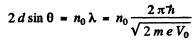where no is an unknown integer/For the next higher voltage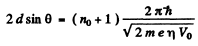Thus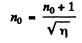or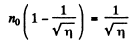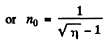Going back we get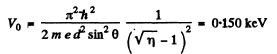Note : In the Bragg’s formula, θ is the glancing angle and not the angle of incidence. We have obtained correct result by taking θ to be the glancing angle. If θ is the angle of incidence, then the glancing angle will be 90 - θ. Then the final answer will be smaller by a factor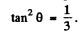Q.61. A narrow beam of monoenergetic electrons falls normally on the surface of a Ni single crystal. The reflection maximum of fourth order is observed in the direction forming an angle θ = 55° with the normal to the surface at the energy of the electrons equal to T = 180 eV. Calculate the corresponding value of the interplanar distance.

Ans. Path  difference is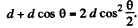Thus for reflection maximum of the kth order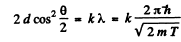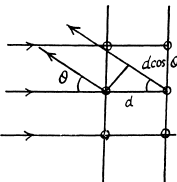Hence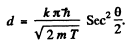substitution with k = 4gives

d = 0.232

Q.62. A narrow stream of electrons with kinetic energy T = 10 keV passes through a polycrystalline aluminium foil, forming a system of diffraction fringes on a screen. Calculate the interplanar distance corresponding to the reflection of third order from a certain system of crystal planes if it is responsible for a diffraction ring of diameter D = 3.20 cm. The distance between the foil and the screen is l = 10.0 cm.

Ans.  See the analogous problem with X - rays (5.156) The glancing angle is obtained from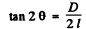where D = diameter of the ring, l = distance from the foil to the screen.
Then for the third order Bragg reflection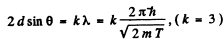Thus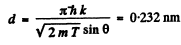Q.63. A stream of electrons accelerated by a potential difference V falls on the surface of a metal whose inner potential is V1 = 15 V. Find:
(a) the refractive index of the metal for the electrons accelerated by a potential difference V = 150 V;
(b) the values of the ratio V/Vt  at which the refractive index differs from unity by not more than η = 1.0%.

Ans. Inside the metal, there is a negative potential energy of - eVi}. (This potential energy prevents electrons from leaking out and can be measured in photoelectric effect etc.) An electron whose K.E. is eV outside the metal w ill find its K.E. increased to e (V + Vi) in th e metal. Then

(a) de Broglie wavelength in the metal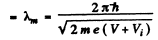Also de Broglie wavelength in vacuum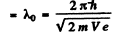Hence refractive index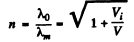Substituting we get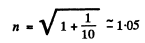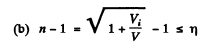then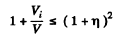or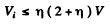or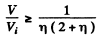For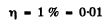we get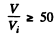Q.64. A particle of mass m is located in a unidimensional square potential well with infinitely high walls. The width of the well is equal to l. Find the permitted values of energy of the particle taking into account that only those states of the particle's motion are realized for which the whole number of de Broglie half-waves are fitted within the given well.

Ans.  The energy inside the well is all kinetic if energy is measured from the value inside. We require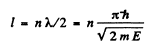or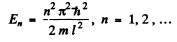Q.65. Describe the Bohr quantum conditions in terms of the wave theory: demonstrate that an electron in a hydrogen atom can move only along those round orbits which accommodate a whole number of de Broglie waves.

Ans. The Bohr condition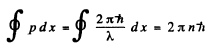For the case when λ is constant (for example in circular orbits) this means

2nr = nλ

Here r is the radius of the circular orbit.

Offer running on EduRev: Apply code STAYHOME200 to get INR 200 off on our premium plan EduRev Infinity!

111 docs

,

,

,

,

,

,

,

,

,

,

,

,

,

,

,

,

,

,

,

,

,

;